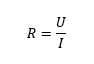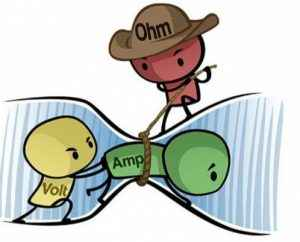FAQ’S

# What is the relationship between voltage, current, and electrical resistance?

The electric voltage (U) and electric current (I) are very different concepts, better known as voltage and current.

It is impossible to refer to electric voltage and electric current without mentioning the law of all laws, the Ohm's law, that has the following relation:Where:
R: Electric resistance, in Ohm (Ω)
U: Electric voltage, in Volts (V)
A: Electric current, in Ampere (A)

Basically, the electrical resistance is the easiness or difficulty that the materials have, to opposed to the electric current passage. When is mentioned that a determined material has good electric resistance, it means that he opposes in a more effective way to the electric currents passage.

To better understand, we can make an analogy with hydraulics. Considering a pipe that carries water, where the voltage corresponds to the water pressure. The current will be the water flow and the electric resistance will be the pipe diameter. In other words, if we increase the pipe diameter (we are reducing the resistance of the water passage) and if we keep the water pressure (voltage) we will get a bigger flow of water (current) passing through the pipe.(Image example from HPAC, 2019)

Learn more about our different types of electric current generators!